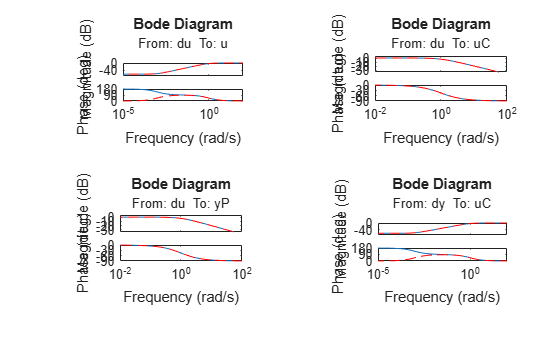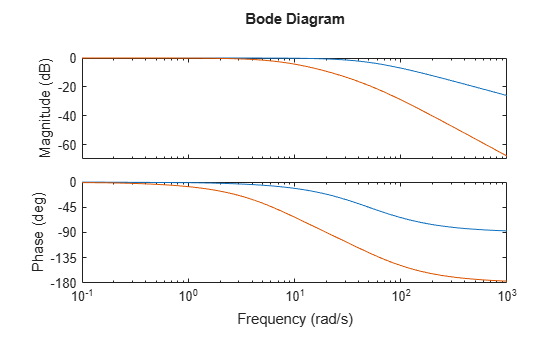# gapmetric

Gap metric and Vinnicombe (nu-gap) metric for distance between two systems

## Syntax

``[gap,nugap] = gapmetric(P1,P2)``
``[gap,nugap] = gapmetric(P1,P2,tol)``

## Description

example

````[gap,nugap] = gapmetric(P1,P2)` computes the gap and Vinnicombe (ν-gap) metrics for the distance between dynamic systems `P1` and `P2`. The gap metric values satisfy 0 ≤ `nugap` ≤ `gap` ≤ 1. Values close to zero imply that any controller that stabilizes `P1` also stabilizes `P2` with similar closed-loop gains.```
````[gap,nugap] = gapmetric(P1,P2,tol)` specifies a relative accuracy for calculating the gaps.```

## Examples

collapse all

Create two plant models. One plant, `P1`, is an unstable first-order system with transfer function 1/(s–0.001). The other plant, `P2`, is stable, with transfer function 1/(s +0.001).

```P1 = tf(1,[1 -0.001]); P2 = tf(1,[1 0.001]);```

Despite the fact that one plant is unstable and the other is stable, these plants are close as measured by the `gap` and `nugap` metrics.

`[gap,nugap] = gapmetric(P1,P2)`
```gap = 0.0021 ```
```nugap = 0.0020 ```

The gap is very small compared to 1. Thus a controller that yields a stable closed-loop system with `P2` also tends to stabilize `P1`. For instance, the feedback controller `C = 1` stabilizes both plants and renders nearly identical closed-loop gains. To see this, examine the sensitivity functions of the two closed-loop systems.

```C = 1; H1 = loopsens(P1,C); H2 = loopsens(P2,C); subplot(2,2,1); bode(H1.Si,'-',H2.Si,'r--'); subplot(2,2,2); bode(H1.Ti,'-',H2.Ti,'r--'); subplot(2,2,3); bode(H1.PSi,'-',H2.PSi,'r--'); subplot(2,2,4); bode(H1.CSo,'-',H2.CSo,'r--');```Next, consider two stable plant models that differ by a first-order system. One plant, `P3`, is the transfer function 50/(s+50), and the other plant, `P4`, is the transfer function [50/(s+50)]*8/(s+8).

```P3 = tf(50,[1 50]); P4 = tf(8,[1 8])*P3; figure bode(P3,P4)```Although the two systems have similar high-frequency dynamics and the same unity gain at low frequency, by the `gap` and `nugap` metrics, the plants are fairly far apart.

`[gap,nugap] = gapmetric(P3,P4)`
```gap = 0.6148 ```
```nugap = 0.6147 ```

Consider a plant and a stabilizing controller.

```P1 = tf([1 2],[1 5 10]); C = tf(4.4,[1 0]);```

Compute the stability margin for this plant and controller.

`b1 = ncfmargin(P1,C)`
```b1 = 0.1961 ```

Next, compute the gap between `P1` and the perturbed plant, `P2`.

```P2 = tf([1 1],[1 3 10]); [gap,nugap] = gapmetric(P1,P2)```
```gap = 0.1391 ```
```nugap = 0.1390 ```

Because the stability margin `b1 = b(P1,C)` is greater than the gap between the two plants, `C` also stabilizes `P2`. As discussed in Gap Metrics and Stability Margins, the stability margin `b2 = b(P2,C)` satisfies the inequality `asin(b(P2,C)) ≥ asin(b1)-asin(gap)`. Confirm this result.

```b2 = ncfmargin(P2,C); [asin(b2) asin(b1)-asin(gap)]```
```ans = 1×2 0.0997 0.0579 ```

## Input Arguments

collapse all

Input systems, specified as dynamic system models. `P1` and `P2` must have the same input and output dimensions. If `P1` or `P2` is a generalized state-space model (`genss` or `uss`) then `gapmetric` uses the current or nominal value of all control design blocks.

Relative accuracy for computing the gap metrics, specified as a positive scalar. If gapactual is the true value of the gap (or the Vinnicombe gap), the returned value `gap` (or `nugap`) is guaranteed to satisfy

|1 – `gap`/gapactual| < `tol`.

## Output Arguments

collapse all

Gap between `P1` and `P2`, returned as a scalar in the range [0,1]. A value close to zero implies that any controller that stabilizes `P1` also stabilizes `P2` with similar closed-loop gains. A value close to 1 means that `P1` and `P2` are far apart. A value of 0 means that the two systems are identical.

Vinnicombe gap (ν-gap) between `P1` and `P2`, returned as a scalar value in the range [0,1]. As with `gap`, a value close to zero implies that any controller that stabilizes `P1` also stabilizes `P2` with similar closed-loop gains. A value close to 1 means that `P1` and `P2` are far apart. A value of 0 means that the two systems are identical. Because 0 ≤ `nugap``gap` ≤ 1, the ν-gap can provide a more stringent test for robustness as described in Gap Metrics and Stability Margins.

collapse all

### Gap Metric

For plants P1 and P2, let ${P}_{1}={N}_{1}{M}_{1}^{-1}$ and ${P}_{2}={N}_{2}{M}_{2}^{-1}$ be right normalized coprime factorizations (see `rncf`). Then the gap metric δg is given by:

`${\delta }_{g}\left({P}_{1},{P}_{2}\right)=\mathrm{max}\left\{{\stackrel{\to }{\delta }}_{g}\left({P}_{1},{P}_{2}\right),{\stackrel{\to }{\delta }}_{g}\left({P}_{2},{P}_{1}\right)\right\}.$`

Here, ${\stackrel{\to }{\delta }}_{g}\left({P}_{1},{P}_{2}\right)$ is the directed gap, given by

### Vinnicombe Gap Metric

For P1 and P2, the Vinnicombe gap metric is given by

`${\delta }_{\nu }\left({P}_{1},{P}_{2}\right)=\underset{\omega }{\mathrm{max}}{‖{\left(I+{P}_{2}{P}_{2}^{*}\right)}^{-1/2}\left({P}_{1}-{P}_{2}\right){\left(I+{P}_{1}{P}_{1}^{*}\right)}^{-1/2}‖}_{\infty },$`

provided that $\mathrm{det}\left(I+{P}_{2}^{*}{P}_{1}\right)$ has the right winding number. Here, * denotes the conjugate (see `ctranspose`). This expression is a weighted difference between the two frequency responses P1() and P2(). For more information, see Chapter 17 of .

### Gap Metrics and Stability Margins

The gap and ν-gap metrics give a numerical value δ(P1,P2) for the distance between two LTI systems. For both metrics, the following robust performance result holds:

arcsin b(P2,C2) ≥ arcsin b(P1,C1) – arcsin δ(P1,P2) – arcsin δ(C1,C2),

where the stability margin b (see `ncfmargin`), assuming negative-feedback architecture, is given by

`$b\left(P,C\right)={‖\left[\begin{array}{c}I\\ C\end{array}\right]{\left(I+PC\right)}^{-1}\left[\begin{array}{cc}I& P\end{array}\right]‖}_{\infty }^{-1}={‖\left[\begin{array}{c}I\\ P\end{array}\right]{\left(I+CP\right)}^{-1}\left[\begin{array}{cc}I& C\end{array}\right]‖}_{\infty }^{-1}.$`

To interpret this result, suppose that a nominal plant P1 is stabilized by controller C1 with stability margin b(P1,C1). Then, if P1 is perturbed to P2 and C1 is perturbed to C2, the stability margin is degraded by no more than the above formula. For an example, see Compute Gap Metric and Stability Margin.

The ν-gap is always less than or equal to the gap, so its predictions using the above robustness result are tighter.

The quantity b(P,C)–1 is the signal gain from disturbances on the plant input and output to the input and output of the controller.

### Gap Metrics in Robust Design

To make use of the gap metrics in robust design, you must introduce weighting functions. In the robust performance formula, replace P by W2PW1, and replace C by ${W}_{1}^{-1}C{W}_{2}^{-1}$. You can make similar substitutions for P1, P2, C1 and C2. This form makes the weighting functions compatible with the weighting structure in the H loop shaping control design procedure used by functions such as `loopsyn` and `ncfsyn`.

 Georgiou, Tryphon T. “On the Computation of the Gap Metric.” Systems & Control Letters 11, no. 4 (October 1988): 253–57. https://doi.org/10.1016/0167-6911(88)90067-9.

 Zhou, K., Doyle, J.C., Essentials of Robust Control. London, UK: Pearson, 1997.